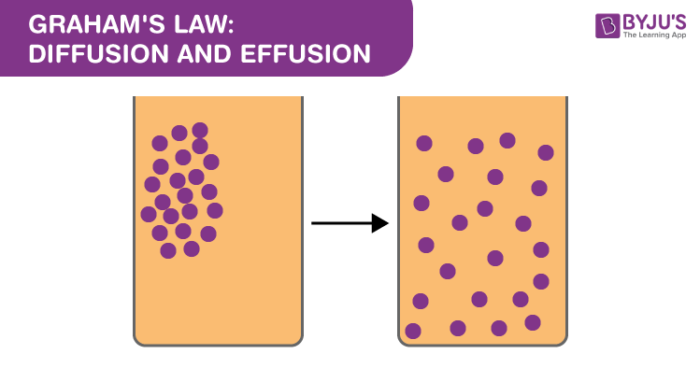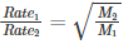Checkout JEE MAINS 2022 Question Paper Analysis : Checkout JEE MAINS 2022 Question Paper Analysis :

# Graham's Law: Diffusion And Effusion

## What is Graham’s law?

Graham’s law states that the rate of diffusion or of effusion of a gas is inversely proportional to the square root of its molecular weight.

Before we discuss Graham’s law in detail, it is appropriate to know the basic definitions of Diffusion and Effusion.

## Diffusion

Diffusion is a phenomenon where there is a movement of one material from an area of high concentration to an area of low concentration. This means particles or molecules spread through the medium. For example, if you spray at one end of the room you would be able to smell it at the other end. This is because of the diffusion phenomenon.## Effusion

Effusion is a process in which air escapes or leaks through a hole whose diameter is considerably smaller than the mean free path of molecules. Under these circumstances, all the molecules which reach the hole will pass through, as collisions between molecules in these places will be negligible.

## Graham’s Law

Graham’s Law which is popularly known as Graham’s Law of Effusion was formulated by Thomas Graham in the year 1848. Thomas Graham experimented with the effusion process and discovered an important feature: gas molecules that are lighter will travel faster than the heavier gas molecules.

According to Graham’s Law, at constant pressure and temperature, molecules or atoms with lower molecular mass will effuse faster than the higher molecular mass molecules or atoms. Thomas even found out the rate at which they escape through diffusion. In other words, it states that the rate of effusion of a gas is inversely proportional to the square root of its molecular mass. This formula is generally used while comparing the rates of two different gases at equal pressures and temperatures. The formula can be written as

$$\begin{array}{l}\frac{Rate_{1}}{Rate_{2}}=\sqrt{\frac{M_{2}}{M_{1}}}\end{array}$$

M1 is the molar mass of gas 1

M2 is the molar mass of gas 2

Rate1 is the rate of effusion of the first gas

Rate2 is the rate of effusion for the second gas

It states that the rate of diffusion or effusion is inversely proportional to its molecular mass.

## Frequently Asked Questions – FAQs

### What is Graham’s law of diffusion?

The principle is that at a given temperature and pressure the rate of diffusion of a gas is inversely proportional to the square root of its density.

### How is Graham’s Law calculated?

Graham’s law states that the rate of diffusion or effusion of a gas is inversely proportional to the square root of its molar mass.

The Formula can be written asM1 is the molar mass of gas 1

M2 is the molar mass of gas 2

Rate1 is the rate of effusion of the first gas

Rate2 is the rate of effusion for the second gas

### Which gas diffuses the fastest?

The rate of effusion for a gas is inversely proportional to the square-root of its molecular mass (Graham’s Law). The gas with the lowest molecular weight will effuse the fastest. The lightest, and therefore fastest, gas is helium.

### What is the value of R in a joule?

The value of R depends on the units involved, but is usually stated with S.I. units as: R = 8.314 J/mol·K. This means that for air, you can use the value R = 287 J/kg·K.

### What is pV = nRT called?

The ideal gas law (PV = nRT) relates to the macroscopic properties of ideal gases.

Take up a quiz on Graham's Law